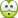# Calculate the inductance of thick solenoid by ansys maxwell

• brahim2016

#### brahim2016

hello, how we calculate the inductance of thick solenoid by ansys maxwell ..!ANSYS Maxwell can calculate the inductance of a thick solenoid using either a 2D or 3D model. First, create a model of the solenoid in ANSYS Maxwell, then set up the parameters for a static analysis. Once the model is set up, use the Magnetic Flux Density results to calculate the inductance of the solenoid. The inductance can be calculated using either the Biot-Savart law or Ampere's circuital law.

## 1. What is a solenoid?

A solenoid is a coil of wire that is tightly wound in a helical shape. It is commonly used in electronic devices to create magnetic fields.

## 2. What is inductance?

Inductance is the property of a circuit or component that resists changes in current flow. It is measured in henries (H) and is represented by the symbol L.

## 3. How is inductance calculated for a thick solenoid?

The inductance of a thick solenoid can be calculated using the formula L = μN²A/l, where μ is the permeability of the material, N is the number of turns, A is the cross-sectional area, and l is the length of the solenoid.

## 4. What is ANSYS Maxwell?

ANSYS Maxwell is a software program used for electromagnetic field simulation. It allows scientists and engineers to analyze and optimize the behavior of electronic and electromechanical devices.

## 5. What are the benefits of using ANSYS Maxwell for calculating inductance?

Using ANSYS Maxwell for calculating inductance allows for accurate and efficient analysis of complex electromagnetic systems. It also provides visualizations of the magnetic fields and allows for optimization of the design to achieve desired inductance values.